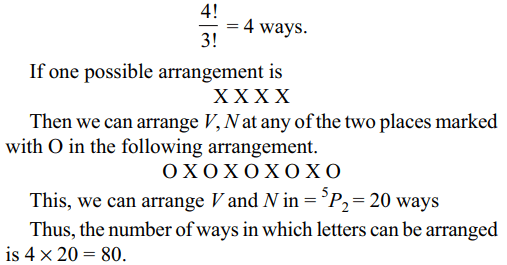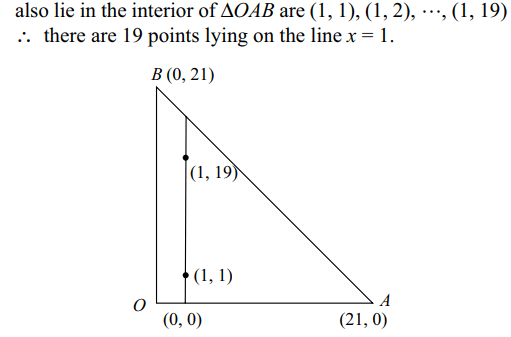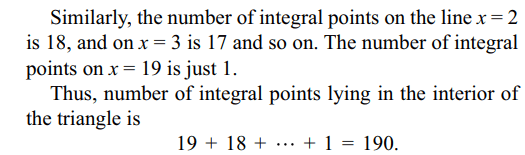## Permutations and Combinations Questions and Answers Part-3

1. The number of subsets of the set $A=\left\{a_{1},a_{2},....a_{n}\right\}$    which contain even number of elements is
a) $2^{n-1}$
b) $2^{n}-1$
c) $2^{n-2}$
d) $2^{n}$

Explanation: For each of the first (n – 1) elements a1, a2,2. The number of ways in which we can post 5 letters in 10 letter boxes is
a) 50
b) $5^{10}$
c) $10^{5}$
d) none of these

Explanation: We can post the first letter in 10 ways, the second letter in 10 ways and so on. Thus, the number of ways of posting 5 letters in 10 letter boxes is 10 * 10 * 10 * 10 * 10 = 105 .

3. The number of five digit telephone numbers having at least one of their digits repeated is
a) 90000
b) 10000
c) 30240
d) 69760

Explanation: The number of five digit telephone numbers which can be formed using the digits 0, 1, 2, .... , 9 is 105 (Refer to explanation of Q2). The number of five digit telephone numbers which have none of their digits repeated is $^{10}P_{5}$ = 30240. Thus, the required number of telephone numbers is 105 – 30240 = 69760.

4. The ten’s digit of 1! + 2! + 3! + .... + 49! is
a) 1
b) 2
c) 3
d) 4

Explanation: We have 1! + 2! + 3! + 4! = 33. Also 5! = 120, 6! = 720, 7! = 5040, 8! = 40320 and 9! = 326880. Thus, the ten digits of 1! + 2! + .... + 9! is 1.
Also, note that n! is divisible by 100 for all n ≥ 10, so that ten’s digit of 10! + 11! + .... + 49! is zero. Therefore, ten’s digit of 1! + 2! + .... + 49! is 1.

5. Four dice are rolled. The number of possible outcomes in which at least one die shows 2 is
a) 1296
b) 625
c) 671
d) 1023

Explanation: The total number of possible outcomes is 64 .
The number of possible outcomes in which 2 does not appear on any die is 54 . Therefore, the number of possible outcomes in which at least one die shows a 2 is 64 – 54 = 1296 – 625 = 671.

6. A set contains (2n + 1) elements. The number of subsets of the set which contain at most n elements is
a) $2^{n}$
b) $2^{n+1}$
c) $2^{n-1}$
d) $2^{2n}$

Explanation: Let N = the number of subsets of the set which contain at most n elements7. A is a set containing n elements. A subset P of A is chosen. The set A is reconstructed by replacing the elements of P. A subset Q of A is again chosen. The number of ways of choosing P and Q so that P $\cap$ Q = $\phi$ is
a) $2^{2n}-^{2n}C_{n}$
b) $2^{n}$
c) $2^{n}-1$
d) $3^{n}$

Explanation: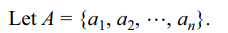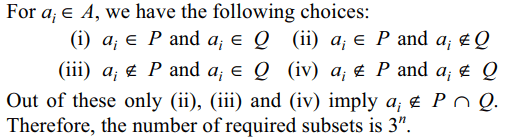8. Let E = {1, 2, 3, 4} and F = {a, b}. Then the number of onto functions from E to F is
a) 14
b) 16
c) 12
d) 32

Explanation: We first find the number of functions from E to F9. The number of ways of arranging letters of the word HAVANA so that V and N do not appear together is
a) 40
b) 60
c) 80
d) 100